##### TI-84 Plus CE Graphing Calculator For Dummies, 3rd EditionHere is a brief review of some of the most important and basic calculator skills you need when you are working on the TI-84 Plus.

## Copying and pasting

Save time by copying and pasting expressions on the Home screen. Press the up-arrow key to scroll through your previous calculations. When a previous entry or answer is highlighted, press [ENTER] to paste it into your current entry line. After you have pasted the expression into the current entry line, you can edit the expression as much as you like.

## Converting a decimal to a fraction

Converting a decimal to a fraction is quick and easy. On the Home screen, press [MATH][ENTER][ENTER]. If you want to convert a fraction to a decimal, press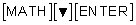or include a decimal point in your calculations.

## Changing the mode

Before you get too far, make sure you can adjust the mode settings on your calculator. The Status bar at the top of the screen indicates some of the most popular mode settings. To see the rest of the mode settings, press [MODE]. Use the arrow keys to highlight the setting you want, and press [ENTER] to select the highlighted setting.

## Accessing hidden menus

Did you know that there are four hidden shortcut menus on your calculator? The four menus are: FRAC (Fraction menu), FUNC (Function menu), MTRX (Matrix menu), and YVAR (Y-variables menu).

To access the FRAC hidden menu, press [ALPHA][Y=]. After pressing [ALPHA], the keys at the top of your keypad become soft keys that activate on-screen menus. Press [ALPHA][Y=] to access the fraction menu and press [ALPHA][WINDOW] to find the math templates. You can access the MTRX shortcut menu only by pressing [ALPHA][ZOOM] (it is not found in standard menus). Finally, press [ALPHA][TRACE] to locate the Y-Var shortcut menu.

## Entering imaginary numbers

You can enter an expression that includes the imaginary number, i, by pressing [2nd][.]. Your calculator automatically simplifies expressions containing imaginary numbers.

Complex numbers cannot be used with the n/d fraction template. Instead, enter complex numbers as fractions using parentheses and the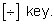## Storing a variable

The letters STO may look like texting language, but the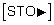key on a calculator is a handy feature. If you plan to use the same number many times when evaluating arithmetic expressions, consider storing that number in a variable. To do so, follow these steps:

1. Enter the number you want to store in a variable.

2. Press3. Press [ALPHA] and press the key corresponding to the letter of the variable in which you want to store the number.

4. Press [ENTER] to store the value.

After you have stored a number in a variable, you can type the variable to insert that number into an arithmetic expression. To do so, press [ALPHA], and press the key corresponding to the letter of the variable in which the number is stored.

## Graphing a function

Entering and graphing functions are on two different screens. Here are the steps to graph a function.

1. Press [Y=] to access the Y= editor.

2. Enter your function.

Press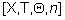to insert an X in the function.

3. Press [ZOOM] to graph the function in a standard viewing window.

Of course, there are many tools that you can use to customize your graph.

## Finding the intersection point

Given two functions, can you find the intersection point? To do so, press [Y=] and enter the functions in Y1 and Y2. Press [ZOOM] to graph both functions. If you can see the point of intersection on the screen, press [2nd][TRACE][ENTER][ENTER][EnTER] to find the point of intersection of the graph.

## Graphing a scatter plot

Graphing a scatter plot is a multistep process:

1. Press [STAT][ENTER] and enter data in lists L1 and L2.

2. Press [2nd][Y=][ENTER] and configure the Plot1 editor.

Highlight ON and press [ENTER]. Then highlight the scatter plot diagram and press [ENTER] to configure the plot editor for a scatter plot.

3. Press [ZOOM] to graph the scatter plot in a ZoomStat window.

## Performing a linear regression

Many different regressions can be performed on data, though the most common regression is a linear regression. To perform a linear regression, follow these steps:

1. Press [STAT][ENTER] and enter data in lists L1 and L2.

2. Tto start the LinReg(ax+b) Wizard, press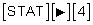3. Configure the wizard and press [ENTER] repeatedly until the regression results appear on the screen.